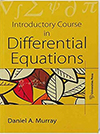## Introductory Course in Differential Equations

#### Price: 595.00

This book provides a brief exposition of some of the devices employed in solving differential equations. Students of physics and engineering will find the clear and concise treatment of the subject, the detailed worked-out examples and the practical applications ideally suited to their need. For students of science, notes in the latter part of the book provide demonstrations of theorems which are referred to, or partially proved, in the first part.

#### Contributors (Author(s), Editor(s), Translator(s), Illustrator(s) etc.)

Daniel A Murray

Chapter 1: Definitions. Formation of a Differential Equation
Chapter 2: Equations of the First Order and of the First Degree
Chapter 3: Equations of the First Order but not of the First Degree
Chapter4: Singular Solutions
Chapter 5: Applications to Geometry, Mechanics and Physics
Chapter 6: Linear Equations with Constant Coefficients
Chapter 7: Linear Equations with Variable Coefficients
Chapter 8: Exact Differential Equations and Equations of Particular Forms. Integration in Series
Chapter 9: Equations of the Second Order
Chapter 10: Geometrical, Mechanical and Physical Applications
Chapter 11: Ordinary Differential Equations with More Than Two Variables
Chapter 12: Partial Differential Equations
Miscellaneous Notes